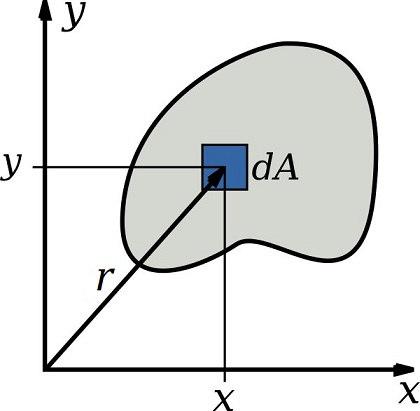# Difference Between Moment of Inertia and Polar Moment of Inertia

## Main Difference – Moment of Inertia vs. Polar Moment of Inertia

Moment of inertia (which refers to the mass second moment of inertia) and polar (second) moment of inertia are both quantities which describe an object’s ability to resist changes due to torques applied to it. The main difference between moment of inertia and polar moment of inertia is that the moment of inertia measures how an object resists angular acceleration, whereas the polar moment of inertia measures how an object resists torsion.

## What is Moment of Inertia

Moment of inertia for a body is defined with the following integral:$I=\int r^2\:\mathrm dm$

where$r$ is the distance of the mass element$\mathrm dm$ from the axis of rotation.

Physically, the moment of inertia gives the ability of a body to resist angular acceleration (analogous to how mass resists linear acceleration) when subject to a torque. If the moment of inertia is large, then the angular acceleration produced by a given torque would be smaller. The unit of moment of inertia is kg m2.

Moment of inertia is used to calculate rotational kinetic energy and also to calculate torque in terms of mass and angular acceleration.

## What is Polar Moment of Inertia

Polar second moment of inertia gives an object’s ability to resist torsion (i.e. “twisting”) about a given axis due to an applied torque. If the polar moment of inertia is large, the torsion produced by a given torque would be smaller. Polar moment of inertia is defined as:$I=\int r^2\:\mathrm dA$

where$r$ is the distance of the area element$\mathrm dA$ from the axis of rotation. The unit of polar moment of inertia is m4.Difference Between Moment of Inertia and Polar Moment of Inertia – Defining Polar Moment of Inertia (here shown in 2D)

Polar second moment of area is often confused with the area second moment of inertia, which is defined:$I=\int x^2\:\mathrm dA$

where$x$ is the distance of the area element$\mathrm dA$ from a specific plane.  This also has units of m4, however physically this quantity indicates the resistance of an object to bend about a certain plane when subjected to a torque.

## Difference Between Moment of Inertia and Polar Moment of Inertia

### Mathematical definition of Moment of Inertia and Polar Moment of Inertia

Moment of inertia is defined as$I=\int r^2\:\mathrm dm$ .

Polar moment of inertia is defined as$I=\int r^2\:\mathrm dA$ .

### Physical Meaning

Moment of inertia is a measurement of an object’s resistance to angular acceleration.

Polar moment of inertia is a measurement of an object’s resistance to torsion (twisting).

### Units

Moment of inertia is measured in units of kg m2.

Polar moment of inertia is measured in units of m4.

###### “A diagram showing the elemental area used in calculating the polar moment of inertia of a flat object.” by Inductiveload (Own work) [Public Domain], via Wikimedia Commons (Modified)